• Core Animation is awesome. It makes doing a lot of complex, fancy animations downright easy. One of the really nice built-in features of C
Core
Animation is awesome. It makes doing a lot of complex, fancy animations downright easy. One of the really nice built-in features of Core Animation is the ability to use animation curves. These curves let you specify whether the animation happens
linearly (at the same pace throughout the animation), or whether the animation eases in, eases out, or does both.
When you have to go closer to the metal and use OpenGL ES, you're not so lucky. We don't have animation curves provided for us in OpenGL ES. We have to interpolate ourselves. Fortunately, the math behind animation curves is straightforward. Plus, there are
far more curves than just the four Apple offers.
I haven't run across a good library for generating animation curves, so I've decided to release my animation curve functions as public domain (no attribute required, no rights reserved). Here is a graph of all the different animation curves I'm releasing: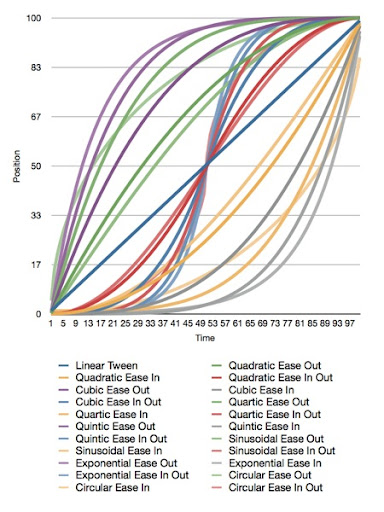Here is the original Numbers.app document that generated the graph, and here is the Xcode
project that generated the data. The project also contains all the functions needed to plot these curves.
Apple doesn't document which calculations they use for easing, but my guess is that they're quadratic. I'm not sure, though, since many of the curves yield similar results.
All of the interpolation functions included in the Xcode project above take three inputs and return a GLfloat containing the interpolated value. The first parameter, t, is
the percent of the way through the animation you want a value calculated for. This is a clamped float that should be in the range 0.0 to 1.0. Values above 1.0 will
be treated as 1.0 and values below 0.0 are treated as 0.0. The second parameter, start, is the value when the animation starts.
The third parameter, end, is the final value to be animated toward.
If you want to apply a curve to a CGPoint or Vector3D, you have to call the function multiple times for each component (x/y or x/y/z).
Have fun!
Here are the functions included in the project above:
#include <OpenGLES/ES2/gl.h>
#include <OpenGLES/ES2/glext.h>
#include <math.h>

#define BoundsCheck(t, start, end) \
if (t <= 0.f) return start;        \
else if (t >= 1.f) return end;

GLfloat LinearInterpolation(GLclampf t, GLfloat start, GLfloat end)
{
BoundsCheck(t, start, end);
return t * end + (1.f - t) * start;
}
#pragma mark -
#pragma mark Quadratic
GLfloat QuadraticEaseOut(GLclampf t, GLfloat start, GLfloat end)
{
BoundsCheck(t, start, end);
return   -end * t * (t - 2.f) -1.f;
}
GLfloat QuadraticEaseIn(GLclampf t, GLfloat start, GLfloat end)
{
BoundsCheck(t, start, end);
return end * t * t + start - 1.f;
}
GLfloat QuadraticEaseInOut(GLclampf t, GLfloat start, GLfloat end)
{
BoundsCheck(t, start, end);
t *= 2.f;
if (t < 1.f) return end/2.f * t * t + start - 1.f;
t--;
return -end/2.f * (t*(t-2) - 1) + start - 1.f;
}
#pragma mark -
#pragma mark Cubic
GLfloat CubicEaseOut(GLclampf t, GLfloat start, GLfloat end)
{
BoundsCheck(t, start, end);
t--;
return end*(t * t * t + 1.f) + start - 1.f;
}
GLfloat CubicEaseIn(GLclampf t, GLfloat start, GLfloat end)
{
BoundsCheck(t, start, end);
return end * t * t * t+ start - 1.f;
}
GLfloat CubicEaseInOut(GLclampf t, GLfloat start, GLfloat end)
{
BoundsCheck(t, start, end);
t *= 2.;
if (t < 1.) return end/2 * t * t * t + start - 1.f;
t -= 2;
return end/2*(t * t * t + 2) + start - 1.f;
}
#pragma mark -
#pragma mark Quintic
GLfloat QuarticEaseOut(GLclampf t, GLfloat start, GLfloat end)
{
BoundsCheck(t, start, end);
t--;
return -end * (t * t * t * t - 1) + start - 1.f;
}
GLfloat QuarticEaseIn(GLclampf t, GLfloat start, GLfloat end)
{
BoundsCheck(t, start, end);
return end * t * t * t * t + start;
}
GLfloat QuarticEaseInOut(GLclampf t, GLfloat start, GLfloat end)
{
BoundsCheck(t, start, end);
t *= 2.f;
if (t < 1.f)
return end/2.f * t * t * t * t + start - 1.f;
t -= 2.f;
return -end/2.f * (t * t * t * t - 2.f) + start - 1.f;
}
#pragma mark -
#pragma mark Quintic
GLfloat QuinticEaseOut(GLclampf t, GLfloat start, GLfloat end)
{
BoundsCheck(t, start, end);
t--;
return end * (t * t * t * t * t + 1) + start - 1.f;
}
GLfloat QuinticEaseIn(GLclampf t, GLfloat start, GLfloat end)
{
BoundsCheck(t, start, end);
return end * t * t * t * t * t + start - 1.f;
}
GLfloat QuinticEaseInOut(GLclampf t, GLfloat start, GLfloat end)
{
BoundsCheck(t, start, end);
t *= 2.f;
if (t < 1.f)
return end/2 * t * t * t * t * t + start - 1.f;
t -= 2;
return end/2 * ( t * t * t * t * t + 2) + start - 1.f;
}
#pragma mark -
#pragma mark Sinusoidal
GLfloat SinusoidalEaseOut(GLclampf t, GLfloat start, GLfloat end)
{
BoundsCheck(t, start, end);
return end * sinf(t * (M_PI/2)) + start - 1.f;
}
GLfloat SinusoidalEaseIn(GLclampf t, GLfloat start, GLfloat end)
{
BoundsCheck(t, start, end);
return -end * cosf(t * (M_PI/2)) + end + start - 1.f;
}
GLfloat SinusoidalEaseInOut(GLclampf t, GLfloat start, GLfloat end)
{
BoundsCheck(t, start, end);
return -end/2.f * (cosf(M_PI*t) - 1.f) + start - 1.f;
}
#pragma mark -
#pragma mark Exponential
GLfloat ExponentialEaseOut(GLclampf t, GLfloat start, GLfloat end)
{
BoundsCheck(t, start, end);
return end * (-powf(2.f, -10.f * t) + 1.f ) + start - 1.f;
}
GLfloat ExponentialEaseIn(GLclampf t, GLfloat start, GLfloat end)
{
BoundsCheck(t, start, end);
return end * powf(2.f, 10.f * (t - 1.f) ) + start - 1.f;
}
GLfloat ExponentialEaseInOut(GLclampf t, GLfloat start, GLfloat end)
{
BoundsCheck(t, start, end);
t *= 2.f;
if (t < 1.f)
return end/2.f * powf(2.f, 10.f * (t - 1.f) ) + start - 1.f;
t--;
return end/2.f * ( -powf(2.f, -10.f * t) + 2.f ) + start - 1.f;
}
#pragma mark -
#pragma mark Circular
GLfloat CircularEaseOut(GLclampf t, GLfloat start, GLfloat end)
{
BoundsCheck(t, start, end);
t--;
return end * sqrtf(1.f - t * t) + start - 1.f;
}
GLfloat CircularEaseIn(GLclampf t, GLfloat start, GLfloat end)
{
BoundsCheck(t, start, end);
return -end * (sqrtf(1.f - t * t) - 1.f) + start - 1.f;
}
GLfloat CircularEaseInOut(GLclampf t, GLfloat start, GLfloat end)
{
BoundsCheck(t, start, end);
t *= 2.f;
if (t < 1.f)
return -end/2.f * (sqrtf(1.f - t * t) - 1.f) + start - 1.f;
t -= 2.f;
return end/2.f * (sqrtf(1.f - t * t) + 1.f) + start - 1.f;
}


展开全文animation graph xcode plot features
• ## IS曲线及其推导

千次阅读 2020-02-16 18:59:41
1．产品市场均衡：产品市场上总供给与总需求相等。 2．产品市场的均衡条件： ...4．IS的含义：描述产品市场达到宏观均衡，即i=s时，总产出与利率之间的关系。 根据凯恩斯的储蓄函数s=s(Q)和投资函数i = i (r)...
1．产品市场均衡：产品市场上总供给与总需求相等。

2．产品市场的均衡条件：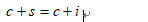简化为：s = i

实际表示的是资本市场均衡的条件，但在封闭的经济中，它又表示产品市场的均衡。

3．均衡的收入的公式：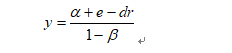均衡的国民收入与利率成反向变化。

4．IS的含义：描述产品市场达到宏观均衡，即i=s时，总产出与利率之间的关系。

根据凯恩斯的储蓄函数s=s(Q)和投资函数i = i (r) 。两部门产品市场均衡条件可写为：r取决于货币市场，这里外生给定；产品市场只能决定产出Q。

5．IS曲线含义：

在资本市场中供给与需求相等时所有实际国民收入和利率的组合。

总产出与利率之间存在着反向变化的关系，即利率提高时总产出水平趋于减少，利率降低时总产出水平趋于增加。处于IS曲线上的任何点位都表示i=s，偏离IS曲线的任何点位都表示没有实现均衡。

6．IS曲线的推导

如下图，图中（a）(c)和(d)是倒置的，各轴是正值。图（a）时表示投资函数，投资和利息率按反方向变化，投资是利息的减函数。（c）表示储蓄函数，s是实际国民收入的增函数，与收入同方向变化。图（b）表示储蓄与投资的均等关系，450线上的任意一点到两轴的距离导相等。图（d）表示商品市场均衡时r与Q的关系。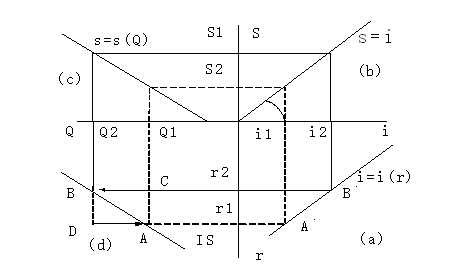首先，从图(a)的投资曲线上任取一点A，表示在利息率为Q1时，投资为i1；

然后由A作连线交于图（b）中的450线。根据s=i 的均衡条件，在投资等于i1时，储蓄应为s1 ；

根据图（c）的储蓄函数，储蓄函数为s1时，实际国民收入为Q1，从而在（d）中找到利息率为Q1、收入为Q1时储蓄与投资均衡点A。

同样的方法，在图(d)中可以得到B点。连接AB两点就可以获得IS曲线。

注意：如果储蓄函数与投资函数不是直线，那就需要选取与投资函数曲线上更多的点所对应的储蓄函数上的收入来做出IS曲线.

7．产品市场的自动均衡机制

1) 例如C、D点都是非均衡点，在C点，是Q1与r2的组合，当利息率为r2时，投资应为i2，而在收入为Q1时，储蓄为s1，从而在Q1与r2时，商品市场是不均衡的，这表现为i2> s1。D点是Q2与r1的组合，当利息率为r1时，投资应为i1，而在收入为Q2时，储蓄为s2，从而商品市场是不均衡的，这表现为i1<s2。

2）商品市场非均衡时的调整

古典学派理论中，当储蓄与投资不平衡时，利息率会发生变动以调整储蓄与投资的不平衡。

在凯恩斯的理论中，利息率完全是货币市场中货币供给与需求决定的，利息率并不能调整储蓄与投资的平衡。因此收入水平必须变动，以使储蓄与投资保持平衡。凯恩斯理论中认为收入是个变量，在储蓄函数给定的情况下，投资水平将决定收入水平，主要是通过存货调节机制实现，这一点决定了在商品市场非均衡条件下的调整方向。

投资大于储蓄，i2 > s1，从而使收入水平会上升，即从Q1移向Q2以使储蓄与投资保持平衡，从而C点会向B点的方向移动。

投资小于储蓄，i1 < s2，从而使收入水平下降，从Q2移向Q1，D点的变动方面是向A点移动。

结论：

如果某一点位处于IS曲线右边，表示i＜s，即现行的利率水平过高，从而导致投资规模小于储蓄规模。如果某一点位处于IS曲线的左边，表示i＞s，即现行的利率水平过低，从而导致投资规模大于储蓄规模。自动调整机制，使得它们回到均衡。

决定投资与储蓄平衡的不是利息率的变动，而是收入的变动，不是储蓄决定投资，而是投资通过收入水平的变动决定储蓄。

由于投资是利息的减函数，而储蓄是收入的增函数，从而随着利息率的下降和投资的增加，收入必须上升，才能保证储蓄与投资的相等。因而为了保持商品市场的均衡，利息率与收入是按相反的方向变动的。


展开全文• <p>I would like to build in a factor to apply to that value (bad affect or questionable affect) that would reduce it to zero affect (in either case) if there is only 1 row of data. so.. some thing ...c math php
• 使用以下公式返回欧拉螺旋参数k，伽玛和曲线长度：曲率K（s）=伽玛* s + k（此处s是圆弧长度） / * @Brief：用于查找欧拉螺旋的参数 between two points given the tangents at the points Since there are ...
• x和y各为9个数，其中y已经是取过自然对数的了，拟合成形如f(x) = a*exp(b*x) + c*exp(d*x)的公式，并提示到：where x is normalized by mean 0.4976 and std 0.3081，请问，这是怎回事？我如何使用这个公式？我试过...数据挖掘
• 欧拉公式求长期率的matlab代码数论Python 实现数论资料的Python模块集合。 Number-Theory-Python软件包当前包括： NumbThy.pdf：数论短期课程 numbthy.py：基本数论函数 gaussint.py：对高斯整数的基本运算 ...
• 看了半天网上的讲解，我也不是很懂，上面的公式 ，大概让我知道了些，原来这平滑的曲线，是用这公式得出的。不研究里面具体的算法了，下面说下在安卓上怎么实现。感谢http://weibo.com/GcsSloop?is_hot=1，我从这里...
原理图：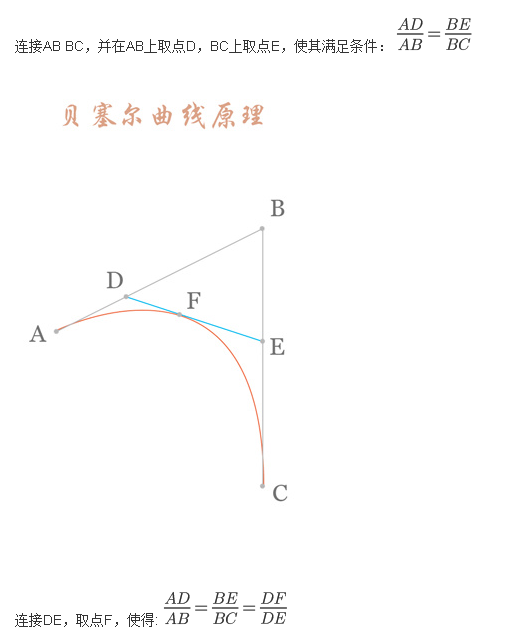看了半天网上的讲解，我也不是很懂，上面的公式 ，大概让我知道了些，原来这平滑的曲线，是用这公式得出的。不研究里面具体的算法了，下面说下在安卓上怎么实现。感谢http://weibo.com/GcsSloop?is_hot=1，我从这里获取的资料。

1.二阶贝塞尔曲线绘制
谷歌封装了一个方法quadTo，给出起点，控制点以及终点，就可以直接绘制二阶贝塞尔取消，demo如下

package com.wx.beziermaker;

import android.content.Context;
import android.graphics.Canvas;
import android.graphics.Color;
import android.graphics.Paint;
import android.graphics.Path;
import android.graphics.PointF;
import android.view.MotionEvent;
import android.view.View;

/**
* Created by huqing on 2016/6/27.
*/
public class TwoBezier extends View {
private Paint mPaint;
private int centerX, centerY;
private PointF start, end, fingerPoint;

/**
*     使用，例：
*    TwoBezier mBezier = new TwoBezier(this);
*    setContentView(mBezier);
*
* @param context
*/
public TwoBezier(Context context) {
super(context);
//画笔
mPaint = new Paint();
mPaint.setStrokeWidth(8);
mPaint.setStyle(Paint.Style.STROKE);
mPaint.setTextSize(60);
//三点确定该二阶曲线
start = new PointF(0, 0);
end = new PointF(0, 0);
fingerPoint = new PointF(0, 0);
}

/**
* 手指触碰时获取当前手指坐标
* @param event
* @return
*/
@Override
public boolean onTouchEvent(MotionEvent event) {
// 根据触摸位置更新控制点，并提示重绘
fingerPoint.x = event.getX();
fingerPoint.y = event.getY();
invalidate();//调用onDraw方法
return true;
}

@Override
protected void onDraw(Canvas canvas) {
super.onDraw(canvas);
// 绘制数据点和控制点
mPaint.setColor(Color.GRAY);
mPaint.setStrokeWidth(20);
canvas.drawPoint(start.x, start.y, mPaint);
canvas.drawPoint(end.x, end.y, mPaint);
canvas.drawPoint(fingerPoint.x, fingerPoint.y, mPaint);
// 绘制辅助线
mPaint.setStrokeWidth(4);
canvas.drawLine(start.x, start.y, fingerPoint.x, fingerPoint.y, mPaint);
canvas.drawLine(end.x, end.y, fingerPoint.x, fingerPoint.y, mPaint);
// 绘制贝塞尔曲线
mPaint.setColor(Color.RED);
mPaint.setStrokeWidth(8);
//绘制曲线路径
Path path = new Path();
//起点
path.moveTo(start.x, start.y);
//关键方法：谷歌封装好的贝塞尔曲线绘制方法quadTo，给出控制点和终点
path.quadTo(fingerPoint.x, fingerPoint.y, end.x, end.y);
canvas.drawPath(path, mPaint);
}

/**
* onSizeChanged的启动时间在onDraw之前,初始化该View时获取到长宽
*/
@Override
protected void onSizeChanged(int w, int h, int oldw, int oldh) {
super.onSizeChanged(w, h, oldw, oldh);
//中心点
centerX = w / 2;
centerY = h / 2;
//左侧点
start.x = centerX - 200;
start.y = centerY;
//右侧点
end.x = centerX + 200;
end.y = centerY;
//控制点，就是手指移动的那个点
fingerPoint.x = centerX;
fingerPoint.y = centerY - 100;
}
}


2.三阶贝塞尔曲线绘制
谷歌封装了一个方法cubicTo，给出起点，控制点以及终点，就可以直接绘制三阶贝塞尔取消，demo如下

import android.content.Context;
import android.graphics.Canvas;
import android.graphics.Color;
import android.graphics.Paint;
import android.graphics.Path;
import android.graphics.PointF;
import android.util.AttributeSet;
import android.view.MotionEvent;
import android.view.View;

/**
* Created by huqing on 2016/6/27.
*/
public class BezierThree extends View {

private Paint mPaint;
private int centerX, centerY;
private PointF start, end, fingerPointOne, fingerPointTwo;
private boolean mode = true;

public BezierThree(Context context) {
this(context, null);
}

public BezierThree(Context context, AttributeSet attrs) {
super(context, attrs);
//画笔
mPaint = new Paint();
mPaint.setColor(Color.BLACK);
mPaint.setStrokeWidth(8);
mPaint.setStyle(Paint.Style.STROKE);
mPaint.setTextSize(60);
//中间点
start = new PointF(0, 0);
end = new PointF(0, 0);
fingerPointOne = new PointF(0, 0);
fingerPointTwo = new PointF(0, 0);
}

@Override
protected void onSizeChanged(int w, int h, int oldw, int oldh) {
super.onSizeChanged(w, h, oldw, oldh);
centerX = w / 2;
centerY = h / 2;

// 初始化数据点和控制点的位置
start.x = 10;
start.y = centerY;
end.x = w - 10;
end.y = centerY;
fingerPointOne.x = centerX;
fingerPointOne.y = centerY - 100;
fingerPointTwo.x = centerX;
fingerPointTwo.y = centerY - 100;
}

@Override
public boolean onTouchEvent(MotionEvent event) {
// 根据触摸位置更新控制点，并提示重绘
if (mode) {
fingerPointOne.x = event.getX();
fingerPointOne.y = event.getY();
} else {
fingerPointTwo.x = event.getX();
fingerPointTwo.y = event.getY();
}
invalidate();
return true;
}

@Override
protected void onDraw(Canvas canvas) {
super.onDraw(canvas);
//        以下是辅助理解的线条和点
//        // 绘制数据点和控制点
//        mPaint.setColor(Color.GRAY);
//        mPaint.setStrokeWidth(20);
//        canvas.drawPoint(start.x, start.y, mPaint);
//        canvas.drawPoint(end.x, end.y, mPaint);
//        canvas.drawPoint(fingerPointOne.x, fingerPointOne.y, mPaint);
//        canvas.drawPoint(fingerPointTwo.x, fingerPointTwo.y, mPaint);
//        // 绘制辅助线
//        mPaint.setStrokeWidth(4);
//        canvas.drawLine(start.x, start.y, fingerPointOne.x, fingerPointOne.y, mPaint);
//        canvas.drawLine(fingerPointOne.x, fingerPointOne.y, fingerPointTwo.x, fingerPointTwo.y, mPaint);
//        canvas.drawLine(fingerPointTwo.x, fingerPointTwo.y, end.x, end.y, mPaint);

// 绘制贝塞尔曲线
mPaint.setColor(Color.RED);
mPaint.setStrokeWidth(8);
Path path = new Path();
//起点
path.moveTo(start.x, start.y);
//贝塞尔曲线中间途径的点
path.cubicTo(fingerPointOne.x, fingerPointOne.y, fingerPointTwo.x, fingerPointTwo.y, end.x, end.y);
canvas.drawPath(path, mPaint);
}
}

展开全文android
• 从http://mathworld.wolfram.com/SphericalHelix.html上找到如下一些关于该曲线的说明,不过似乎他的公式和我的脚本完全是两个东西.. The tangent indicatrix of a curve of constant precession is a ...
从http://mathworld.wolfram.com/SphericalHelix.html上找到如下一些关于该曲线的说明,不过似乎他的公式和我的脚本完全是两个东西..
The tangent indicatrix of a curve of constant precession is a spherical helix. The equation of a spherical helix on a sphere with radiusmaking an anglewith the z-axis is(1)(2)(3)

The projection on the-plane is an epicycloid with radii(4)(5)

#http://www.mathcurve.com/courbes3d/helicespheric/helicespheric.shtml

vertices = 12000

t = from 0 to (80*PI)

k = rand2(0.0, 1)

s = sin(t)
c = cos(t)

a = sin(k*t)
b = cos(k*t)

r = 10

x = r*(k*c*b - s*a)
z = r*(k*s*b + c*a)
y = r*sqrt(1 - k*k)*b`
展开全文• 完成了连铸和模铸LZ50车轴钢疲劳裂纹扩展试验, 得到了两类LZ50车轴钢的疲劳裂纹扩展曲线。采用Par is公式和另一种全范围表达式研究了两种铸造工艺下LZ50车轴钢的疲劳裂纹扩展规 律
• MATLAB GUI设计学习手记进度条绘图进度图片添加文字使用patch颜色数据说明编辑公式简易时钟交互曲线设置小结 原书见 https://www.ilovematlab.cn/thread-568839-1-1.html ... 进度条 ... %清空基本工作空间的变量 ...MATLAB GUI
• 对于不熟悉Mathcad（由Mathsoft公司开发，注意不要与Matlab或Mathematica混淆）的人来说，它可以视为一种通用的数学分析工具，带有WYSIWYG（What You See Is What You Get, 所见即所得）的公式录入，数字化和符号化...
• 这时如果我们输入“12”，就会在单元格中显示“正数：（$12.00）”，如果输入“-0.3”，就会在单元格中显示“负数：（$0.30）”，如果输入“0”，就会在单元格中显示“零”，如果输入文本“this is a book”，就会在...excel
• 数学基础研究基本数学概念（数、几何形状、集合、函数...），及其 如何构成更复杂结构和概念的 层次结构，特别是一类 也被称为元数学概念的 基础性重要结构，用它们来形成数学语言（公式、理论、以及它们的 用来表意...
• # Just make sure you use sufficiently small dt (dt is change in time between steps) for t in time: F_x = 0.0 F_y = 0.0 if (bouyancy == True): F_y = F_y + bouy if (drag == True): F_y = F_y - ...
• Abstract: The Newton iteration method is "Numerical analysis" in this curriculum an important computational method and the thought.The method thought computation equation in the study which this time ...
• , [error term is the value needed to correct for a prediction error between the observed and predicted value] => y=a+y= a+ b1x1+ b2x2+....+e, for multiple independent variables. 在一个线性...算法
• 3.求出样本图像的特征点坐标和测试图像的特征点坐标，找出这两坐标矩阵的H变换公式（利用RANSAC算法），将H变换公式对right图像做透视变换，得到拼接后的右边图像 4.将left原图赋给result对应的ROI区域，大功告成。
• * 高中的数学公式咋都忘了涅，写出来 * 正弦型函数解析式：y=Asin(ωx+φ)+b * 各常数值对函数图像的影响： * A：决定峰值（即纵向拉伸压缩的倍数） * b：表示波形在Y轴的位置关系或纵向移动距离（上加下...php
•  rainDrop.x rainDrop.xLength : rainDrop.x - rainDrop.xLength这是因为，我们的雨滴是一直往下移动即y是增加的，我们上面知道斜率公式为：k=(y1-y2)/(x1-x2)即y1-y2肯定是大于0的，因此当斜率小于0的时候，...开源项目
• 3.2.4 通过输入一个新的SERIES公式添加一个新系列/58 3.3 删除图表系列/58 3.4 为图表系列更改数据区域/59 3.4.1 使用高亮区域更改系列数据/59 3.4.2 通过“选择数据源”对话框来更改系列数据/61 3.4.3 编辑SERIES...
• 3.2.4 通过输入一个新的SERIES公式添加一个新系列/58 3.3 删除图表系列/58 3.4 为图表系列更改数据区域/59 3.4.1 使用高亮区域更改系列数据/59 3.4.2 通过“选择数据源”对话框来更改系列数据/61 3.4.3 编辑SERIES...
• If Not LastSelect_Menu Is Nothing Then With LastSelect_Menu .Picture = LoadPicture() .BackStyle = fmBackStyleTransparent .BorderStyle = fmBorderStyleNone End With End If End Sub '********本模块...